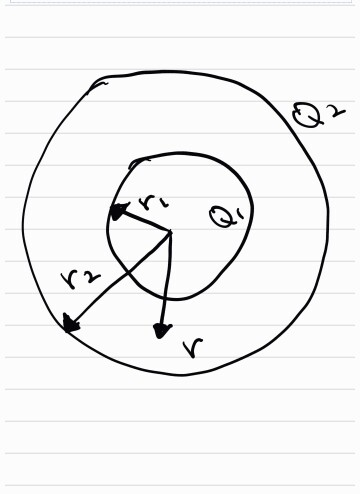# Problem: Two concentric, spherical conducting shells have radii r1 and r2 and charge Q1 and Q2, as shown below.Let r be the distance from the center of the sphere.Finda) from the electric field obtained, determine the electric potential in the region r&gt;r2 and r2&gt;r&gt;r1.

###### FREE Expert Solution

The electric potential due to charge, q is:

$\overline{){\mathbf{V}}{\mathbf{=}}\frac{\mathbf{k}\mathbf{q}}{\mathbf{r}}}$

For r > r2

Let's take the net charge at the center of the concentric shells to be Q1 + Q2

86% (74 ratings)###### Problem Details

Two concentric, spherical conducting shells have radii r1 and r2 and charge Q1 and Q2, as shown below.
Let r be the distance from the center of the sphere.
Finda) from the electric field obtained, determine the electric potential in the region r>r2 and r2>r>r1.

Frequently Asked Questions

What scientific concept do you need to know in order to solve this problem?

Our tutors have indicated that to solve this problem you will need to apply the Electric Potential concept. You can view video lessons to learn Electric Potential. Or if you need more Electric Potential practice, you can also practice Electric Potential practice problems.